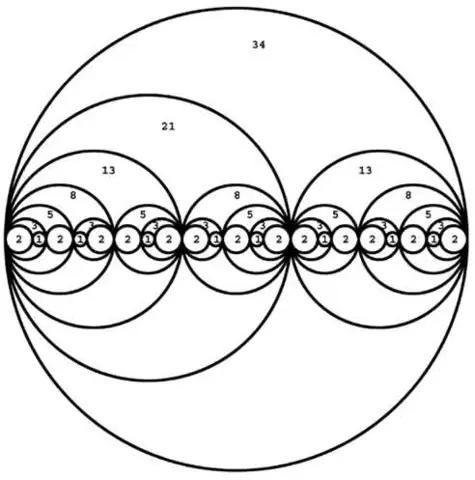top of page
Search

# Cubistic matrix, Torus, and the doubling sequence

Updated: Apr 11, 2021

The side view of a 2D torus is two circles contained within a bigger circle and we can fractal this geometry so the smaller circles also contain two smaller circles and this forms the doubling sequence if we continue this process and it also forms the ying-yang of the I ching and as we saw in Kabbalah holon this sacred geometry fractal which also, therefore, links into the kabbalistic tree of life looks similar to the vibration pattern shown in the same post meaning it encodes all the frequencies/harmonics and therefore all the dimensions also as i said there is a connection between the tree of life and this geometry which makes sense because the tree of life is the tetractys and the hexagonal yod of the tetractys encodes all the vibrational dimensions. The vibrational dimensions are the Kathara grid which forms the doubling sequence. (The torus is a double seed of life which is made up of 12 circles 13 if you include the center one and 13 is the number of spheres that make up the VE which is a 3D seed of life and it fractals to form the 64 tetrahedron grid and as we know toruses are formed out of the 64 tetrahedron fractal also each seed of life is a torus and the flower of life is made up of these toruses so we could replace each circle with this 2D torus)The Fibonacci spiral/numbers forms the doubling sequence which forms a triangle which encodes all the vibrational dimensions and this triangle can be a tetractys also the two circles can be yods in the hexagonal yod and they could be the structure of the hexagon which join 1, 2, 4, 8, 7 and 5. The 24 repeating Fibonacci numbers create Metatrons cube and numbers are fractals of consciousness. The Fibonacci numbers can be turned into this fractal and this forms a base 3 pattern but with no 0's as shown on the right. When we double the Fibonacci spiral we form a star tetrahedron which encodes all frequencies/harmonics.Pascals triangle forms the doubling sequence because each row equals a power of 2 and the powers of 2 form binary as we will explain later. The Kathara grid doubles and when it does it forms a spiral which forms the doubling sequence and this corresponds to the tetractys and the dimensions.

The I Ching uses a complex binary code in its formation of hexagrams. Yin is notated as a broken line while Yang is notated as an unbroken line. These lines are then used in a set of three to form eight trigraphs, which combine to create 64 hexagrams.

The 64 tetrahedron grid is constructed out of 64 tetrahedrons which are constructed out of the 64 hexagrams meaning the 64 tetrahedron grid is made out of binary. This is interesting because it seems like the universe is a simulation but it is not a simulation is just based on numbers. This corresponds the 64 tetrahedron grid to the tetractys and dimensions.

The hexagonal yod is the fingerprint of god which is 124875 and the doubling sequence can be reduced to 124875. Base 2 is binary which is zeros and ones and is formed out of the doubling sequence 1, 2, 4, 8, 16, 32, 64, 128, 256, 512...(powers of 2) and the doubling sequence forms the Kathara grid/cubistic matrix and as we know the cubistic matrix is base 3 because it grows out of the 3×3×3 cube so base 2 is formed out of base 3 but how does base 2 come out of base 3? Well in quantum physics quantum computers run on Qbits which are binary but this binary has 3 states which are 1, 0 and the quantum state where it is 1 and 0 at the same time so this shows how the trinity is formed out of binary.